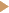# SQL Tutorial

Previous | Home  | Next

### SQL KeywordsIntroductionFROM, SELECT, WHERE, DISTINCTORDER BYAGGREGATE FUNCTIONSGROUP BYHAVINGINNER JOINLEFT JOIN

### ExamplesA structured approach to writing queriesExample 1Example 2Example 3Example 4Example 5Example 6

EXAMPLE 6 : Write a query which can select the countries in which 2 or more persons have been born. Display the name of the country and the number of persons that were born in that country.

 1. Which table(s) contains the information that you need? The exercise contains the noun "persons" which indicates that we need to use the table PERSON. The exercise also requires us to display the names of the countries. Recall from the explanation of example 4 above that if we want to see the names of countries, and not just the country codes, we must also include the table COUNTRY which contains these country names. So, in these query, we need to JOIN tables. As has been discussed, this involves two actions: (1) mentioning the tables that you want to join, after FROM and (2) explaining in the WHERE clause of the query how these two tables should be linked. This is normally according to the formula foreign key = primary key. The result of this step should be : FROM PERSON, COUNTRY WHERE COUNTRY_BORN = COUNTRY_CODE. The resultant table of this statement should be the same as the table that was included above in the explanation of example 4. 2. Are you interested in all the records in this/these table(s)? The exercise appears to imply a criterium: only those countries in which two or more people have been born should be displayed. Nevertheless, this criterium is different from the one that we have seen in example 2. As has been explained, WHERE can only affect a single row in the table. On the basis of only a single record, you can never decide if two or more persons have been born in that same country. Clearly, you need to divide the table into groups first, count the number of rows in each separate group, and then select those groups which contain two or more records. The condition can only be included after the GROUP BY command has divided the table into separate groups. 3. Are you interested in the actual contents of the records or in statistical information about the records in the table? If so, do you want to receive one answer for the entire table, or would you like to receive different answers for different types of records? Thus, the basic principle here is that, if you want to be able to filter a row simply on the basis of its attributes, you need to use WHERE. If you want to get rid of certain groups, on the basis of the outcome of an SQL function, you have to use HAVING. HAVING focuses on a group as a whole, and can thus be used ONLY if you also use GROUP BY. To divide the tables PERSON, CITY into groups on the basis of the city, you need to use FROM PERSON, COUNTRY WHERE COUNTRY_BORN = COUNTRY_CODE GROUP BY COUNTRY_NAME. We choose the column COUNTRY_NAME here and not COUNTRY_CODE because, as has been explained in the previous example, only columns which are mentioned after GROUP BY can be included in the select list. The names are more meaningful than the codes. The result of this will be that the table is divided into a number of different groups, and the number of rows will be calculated for each group.Next, similar to the way in which you can filter rows using the WHERE statement, HAVING will filter groups. You use HAVING to test each group according to a certain criterium. In the HAVING section, you can make use of the same sort of statements as in the WHERE section. The only difference is that, in the case of HAVING, you can also use the SQL functions. In this exercise, we want to select only those groups which contain 2 or more rows. So we need to use FROM PERSON, COUNTRY WHERE COUNTRY_BORN = COUNTRY_CODE GROUP BY COUNTRY_NAME HAVING COUNT(*) >= 2 4. Which columns do you want to see? The number of columns that you can select in queries which contain GROUP BY is highly restricted. You can only use the columns that you mention after GROUP BY and the aggregate function for each group. The select list should be SELECT CITY_NAME, COUNT(*). 5&6 The last two steps can be skipped in the case of this query.

[ up  | next ]